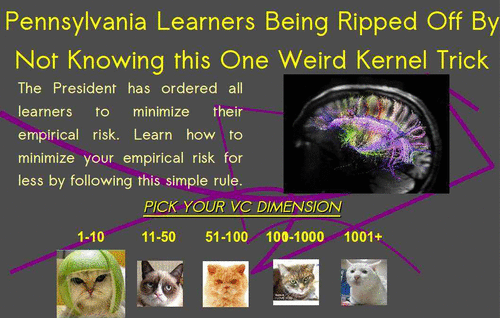# (Reproducing) kernel tricksWARNING: This is very old. If I were to write it now, I would write it differently, and specifically more pedagocgically

Kernel in the sense of the “kernel trick”. Not to be confused with smoothing-type convolution kernels, nor the dozens of related-but-slightly-different clashing definitions of kernel; those can have their own respective pages. Corollary: If you do not know what to name something, call it a kernel.

We are concerned with a particular flavour of kernel in Hilbert spaces, specifically reproducing or Mercer kernels . The associated function space is a reproducing Kernel Hilbert Space, which is hereafter an RKHS.

Kernel tricks comprise the application of Mercer kernels in Machine Learning. The “trick” part is that many machine learning algorithms operate on inner products. Or can be rewritten to work that way. Such algorithms permit one to swap out a boring classic Euclidean definition of that inner product in favour of a fancy RKHS one. The classic machine learning pitch for trying such a stunt is something like “upgrade your old boring linear algebra on finite (usually low-) dimensional spaces to sexy algebra on potentially-infinite-dimensional feature spaces, which still has a low-dimensional representation.” Or, if you’d like, “apply statistical learning methods based on things with an obvious finite vector space representation ($$\mathbb{R}^n$$) to things without one (Sentences, piano-rolls, $$\mathcal{C}^d_\ell$$).”

Mini history: The oft-cited origins of all the reproducing kernel stuff are . It took a while to percolate into random function theory as covariance functions. Thence the idea arrived in statistical inference and signal processing , and now it is ubiquitous.

Practically, kernel methods have problems with scalability to large data sets. To apply any such method you need to keep a full Gram matrix of inner products between every data point, which needs you to know, for $$N$$ data points, $$N(N-1)/2$$ entries of a symmetric matrix. If you need to invert that matrix the cost is $$\mathcal{O}(N^3)$$, which means you need fancy tricks to handle large $$N$$. Fancy tricks depend on what the actual model is, but include Sparse GPs, random-projection inversions, Markov approximations and presumably many more

I’m especially interested in the application of such tricks in

1. kernel regression
2. wide random NNs
3. Nonparametric kernel independence tests
4. Efficient kernel pre-image approximation
5. Connection between kernel PCA and clustering Turns out not all those applications are interesting to me.

## IntroductionsFeature space

There are many primers on Mercer kernels and their connection to ML. Kenneth Tay’s intro is punchy. See , which grinds out many connections with learning theory, or , which is more narrowly focussed on just the Mercer-kernel part which emphasises topological and geometric properties of the spaces, or for an approximation-theory perspective which does not especially concern itself with stochastic processes. I also seem to have bookmarked the following introductions .

Alex Smola (who with, Bernhard Schölkopf) has his name on an intimidating proportion of publications in this area, also has all his publications online.

## Non-scalar-valued “kernels”

Extending the usual inner-product framing, Operator-valued kernels, , generalise to $$k:\mathcal{X}\times \mathcal{X}\mapsto \mathcal{L}(H_Y)$$, as seen in multi-task learning.

### No comments yet. Why not leave one?

GitHub-flavored Markdown & a sane subset of HTML is supported.